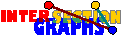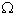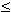# Some Definitions

Formally a graph is a pair (V,E) where V is any set, called the vertex set, and the edge set E is any subset of the set of all 2-element subsets of V. Usually the elements of V, the vertices, are illustrated by bold points or small circles, and the edges by lines between them.
Families of sets (Sx/xV) are called hypergraphs. The sets Sx are called the hyperedges, whereas the elements of the union A of all sets Sx, xV, are the points or vertices of the hypergraph.
The intersection graph(H) of a hypergraph H=(A, (Sx/ xV)) has V as vertex set, and two distinct vertices x,y are joined by an edge whenever SxSy is nonempty.
Look on an example of a hypergraph and its intersection graph.
For every 0-1 matrix we get a row-intersection graph whose vertices correspond to the rows, and where two distinct vertices are adjacent if there is some column where the corresponding rows both have a `1´. The intersection graph of a hypergraph is just the row-intersection graphs of the corresponding adjacency matrix.
By boxes we mean generalized rectangles with sides parallel to the coordinate axes, i.e. subsets {(x1, ... , xd) all aixibi}.
By cliques we mean inclusion-maximal complete subgraphs.
The clique hypergraph of a graph has the same vertices than the graph, and all cliques as hyperedges.
The clique graph of a graph G is the intersection graph of the set of all cliques of G (i.e. the intersection graph of the clique hypergraph of G).
Clique-Helly graphs are graphs whose clique hypergraphs obey the Helly-property
Three vertices form an asteroidal triple whenever any two of them are connected by a path avoiding the third and its neighbors.
Three edges form an asteroidal triple if any two are connected by a path avoiding vertices and neighbors of the third edge.
A class H of hypergraphs is closed under subhypergraphs if every hypergraph obtained from a member of H by deleting some hyperedges is again in H.
an induced subgraph of a graph is obtained by deleting some vertices and all edges incident with one or two of the deleted vertices.
A class G of graphs is closed under subgraphs if every induced subgraph of any member of G is again in G.
A biclique is any inclusion-maximal induced complete bipartite subgraph (where it is even allowed that one partition class is empty).
Dicliques are ordered variants of bicliques.
The complement --G of the graph G has the same vertex set than G, but two distinct vertices are adjacent in --G if and only if they are nonadjacent in G}
The square and the 2-step graph of the graph G have both the same vertex set than G. Two distinct vertices are adjacent in the square if their distance in G is at most 2, and in the 2-step graph if they have some common neighbor in G.

Erich Prisner